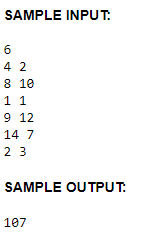# Splitting the field

This is the link of the problem:
http://www.usaco.org/index.php?page=viewproblem2&cpid=645

I still don’t understand why the example output is 107 there, I solve it by hand and get it 114.
So could anyone please explain to me why it’s 107 not 114
Maybe I just get confused or misunderstood
I would be appreciated if someone help me. Thanks

How did you get 107?

I think they are getting 114 when they are supposed to be getting 107.

For the example test:
6 index
4 2 1
8 10 2
1 1 3
9 12 4
14 7 5
2 3 6

First, I would calculate the total area of all these coordinates by getting the x1,x2,y1,y2 (xMin,xMax,yMin,yMax)
x1=1
y1=1
x2=14
y2=12
So the total area to contain these cows are T = (14-1+1)*(12-1+1)= 168

Second, I try to make 2 groups of cows which all the cows have the least distance
G1: index 1,6,3
G2: index 2,4,5
In each groups:
G1: x1=1, y1=1, x2=4, y2=3
area: T1 = (4-1+1)(3-1+1) = 12
G2: x1=8, y1=14, x2=7, y2=12
area: T2 = (8-7+1)
(3-1+1) = 12
The total amount area left that will be saved is: T - (T1 + T2) = 168 - (12 + 42) = 114
I hope this will explain

(cows on the boundary are allowed).

Doesn’t the sample output for the problem say `107`?Yeah? That’s the answer if you allow cows on the boundaries.

1 Like

Oops, I replied to the wrong person above.